Python’s easy readability makes it one of the best programming languages to learn for beginners. A good example of this can be seen in the for loop. While similar loops exist in virtually all programming languages, the Python for loop is easier to come to grips with since it reads almost like English.

In this tutorial, we’ll cover every facet of the for loop and show you how to use it using various examples. We’ll also go into a few common Python commands and functions like join, argv, etc. For more in-depth lessons on for loop and other Python programming concepts, check out this course to learn Python from scratch.

Before We Begin: What You Should Know

To use for loops, you’ll need to be familiar with basic Python concepts. Chiefly, you should be able to:

• Declare variables and create lists.
• Use simple commands like print and return
• Have a basic understanding of if-else statements
• Know how to save and run Python programs

All examples used in this tutorial were written on a Windows machine, though you shouldn’t have any issues using them on OS X or Linux, provided you have Python installed (you can grab a copy here).

How to Use this Tutorial

The only way to master programming is through active participation. Thus, while we’ll introduce you to the core concepts and syntax of the for loop, you must type in each example by hand and take an active interest in learning about the many different commands and functions used throughout this tutorial.

With that out of the way, let’s get started with for loops!

What is a For Loop?

A for loop is used to repeat a piece of code n number of times. The for loop is usually used with a list of things. The basic syntax for the for loop looks like this:

```for item in list:
print item```

Translated into regular English, this would be: “For each item that is present in the list, print the item”.

There are a few things to note here:

• Every for loop must reference a list or a range.
• Every for loop must close with a colon.
• Code to be executed as part of the for loop must be indented by four spaces (or one press of the Tab key).
• To use individual items in the list or range, we have to create a variable (item in the syntax above). There’s no need to declare this variable before creating the for loop. You can also reuse the same variable for other for loops.

You’ve used for loops extensively in your own life. As an example, suppose you have to go grocery shopping. You walk into the grocery store, grab a cart, and pull out your shopping list. As you walk through the aisles, you pull out each item on the shopping list and place it into the cart. In a way, you are using a for loop – for every item present in your shopping list, you pull it out and keep it in your cart.

But enough theory. Let’s see how the for loop works with an actual example:

Example 1

```x = [2, 8, 512] #Creating a list
for i  in x: #Here, i  is the variable used to refer to individual items in the list
print i```

Here’s the output: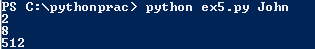Let’s look at another example using range:

Example 2

```for i in range (0, 10):
print i```

The output: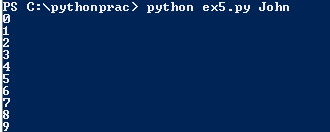You can see how the above example can be used to print a list of numbers within a specified range. You can also do some simple mathematics and print out lists of even or odd numbers, as we’ll see in later examples.

For Loop vs. While Loop

Python supports two kinds of loops – for and while. They are quite similar in syntax and operation, but differ in one crucial aspect: a while loop will run infinitesimally as long as the condition is being met.

A while loop has the following syntax:

```                while condition:
Do something```

Here’s an example:

```                i = 0
while i < 100:
i = i + 1 #This statement is required to make the while loop condition false
print i```

Here’s the output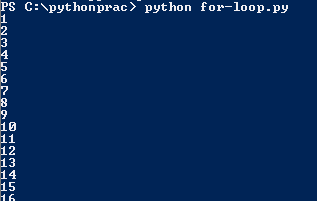If, however, you were to remove the ‘i = i + 1’ part, the while condition will never be fulfilled, i.e. number will never reach 100. Thus, the loop will keep on running endlessly and the program will crash. This is called an infinite loop.

Try it right now – remove the i = i + 1 part and run the program. You should see something like this:Because while loops run infinitesimally until the condition becomes false, they can often cause programs to throw off errors or crash. This is the reason why you should try to use for loops instead of while loops whenever you can.

For Loops and Lists

The most common use of for loops is to extract information from a list.

A list, as you might know, is basically a collection of items or data. A list is essentially just one of the six different types of sequences used in Python. For this tutorial, however, we’ll focus only on two types of sequences: lists and tuples.

The basic syntax of a list is as follows:

`                list_name = [list_item_1, list_item_2, list_item_3]`

Essentially, a list is just like a variable that holds multiple pieces of data. Let’s consider an example:

`                odd_numbers = [1, 3, 5, 7, 9, 11, 13]`

It can hold text strings as well:

`                names = [“John”, “Roger”, “Ben”, “George”]`

Text strings and numbers can go in the same list:

`                names_numbers = [“John”, 1, “Ben”, 2, “George”, 3]`

Printing the above lists will produce the list in its entirety: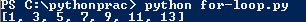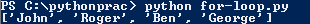Tuples

A tuple is like a list with immutable elements, i.e. the contents of a tuple can’t be changed. Instead of a square bracket like normal lists, tuples sit inside round brackets (). The basic syntax is as follows:

`                tuple_name = (tuple_item_1, tuple_item_2, tuple_item_3)`

Like lists, tuples can hold text strings, numbers or both:

```                even_tuple = (2, 4, 6, 8, 10)
names_tuple = (“John”, “Ben”, “George”)
names_and_numbers = (“John”, 1, “Ben”, 2, “George”, 3)```

Like lists, printing a tuple replicates the tuple in its entirety: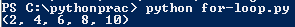But what if you want to print individual items in the list or tuple?

This is where the for loop comes in handy.

The for loop loops over individual items in the list and reproduces the results. You can not only print, but also perform mathematical operations on list items, and add/delete items.

Let’s look at a few examples to understand how this works:

Printing items from list

```list1 = [1, 3, 5, 7, 9, 11]
for item in list1:
print item```

This will print out each individual item in the list: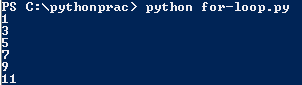It’s the same process for a tuple:

```for i in (1, 2, 3, 4, 5, 6):
print i```

The output: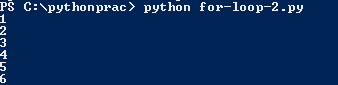Modifying items in list

We can modify list items by performing mathematical operations on them. Below, we’ll take the list of odd numbers above and add 1 to each number:

```list1 = [1, 3, 5, 7, 9, 11]
for item in list1:
item = item + 1
print item```

Note: Instead of writing ‘item = item + 1’, we can use the shorthand, ‘item += 1’.

The result: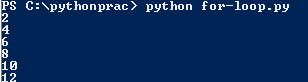What if you want to add items to the list? For this, we’ll use the append() method.

Here’s an example:

```                list1 = [1, 2, 3, 4, 5, 6, 7]
list2 = [8, 9, 10]
list1.append(list2)```

When we print list1, however, we see the following output: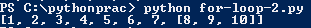Can you spot the problem? The append() method treats all individual items in list2 as one single item. Hence, instead of 8, 9, 10 being treated as separate list items, they get clubbed together.

You can see this if you print just eight element of the list by using print – [8, 9, 10] are treated as a single element.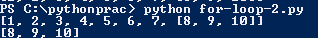So how do we add list items individually?

By using for loops, of course!

```list1 = [1, 2, 3, 4, 5, 6, 7]
list2 = [8, 9, 10]
for i in list2: #Iterating through individual elements in list2
list1.append(i) #Appending these individual elements to list1
print list1```

This is what list1 looks like now: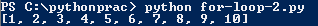Thus, instead of appending the entire list, we’re going through all the elements in list2 separately and adding them to list1. This is just one of the many uses of for loops and lists.

Note: You can add individual elements to the list by using the extend() method as well.

Working with multiple lists

So far, we’ve used for loops with single lists. But what if we wanted to work with multiple lists – that is, a list within a list?

In this case, we’ll have to use nested for loops. We’ll cover nested loops in detail later. For now, we’ll illustrate how to work with multiple lists with an example:

Let’s say we have a list with names, numbers, and names of countries:

`list1 = [["Matt", "Ben", "Jerry"],[1, 2, 3, 4, 5], ["Denmark", "England", "Scotland"]]`

If we use our normal for loop method for printing list items, we get the following result:

```for i in list1:
print i```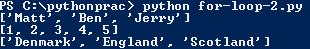But since we want to print individual items from within these lists, we’ll have to use another for loop, like this:

```for i in list1:
for x in i:
print x```

This essentially means that you’re instructing Python to go through the list twice. The output looks like this: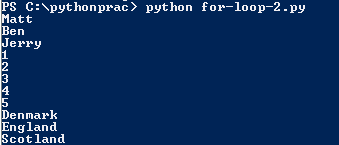We’ll come back to nested for loops later. For now, you should know that to work with lists within lists, you need multiple for loops.

Using Loops with Range

The built-in range() method is especially used when using loops. The range() method basically defines the range through which a particular action must be performed.

As always, the best way to understand this is through an example:

Let’s say we wanted to print the first 10 numbers. The easiest way to do this is using a for loop and the range() method:

```         for i in range(0,10):
print i```

The output: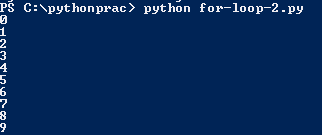The range() method should include both an upper and lower range. In case you use only one value (say, for i in range(10)), Python will automatically assume that the count starts from 0. It is good practice to include both lower and upper range in your code.

Note that the upper bound of the range is not inclusive, i.e. in the above example, 10 would not be included.

But what if you wanted to count backwards? Surely, you would have to pull off some complex programming sorcery to pull it off?

Thankfully, the range() method in Python comes built-in with a feature called step. This is the third argument that can be used with range(). It isn’t necessary, but can be very useful when used right.

Let’s see how it works with an example:

```        for i in range(10, 0, -1):
print i```

Here, 10 is the starting point, 0 is the end point, and -1 is the step. Thus, the count will decrease from 10 to 0.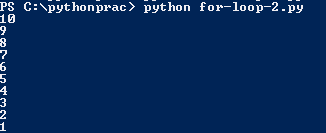We can even count to negatives:

```       for i in range(10, -10, -1):
print i```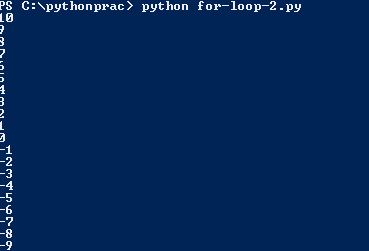The step can be anything. Let’s use it to count all the even numbers from 0 to 20:

```        for i in range(0, 20, 2):
print i```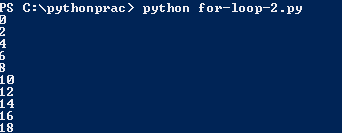The range() will come in very handy when working with numbers. Try to play around with it and see what other kind of sequences you can come up with.

Using For Loops with If-Else Conditional Statements

We’ve seen how useful for loops can be, but you don’t really unleash the power of these loops until you use them with conditional statements like if-else.

If-else statements help the programmer make decisions and guide the program’s flow. To refresh your memory, here’s what the most basic if-else statement looks like:

```                if condition1 = True:
do something
elif condition2 = True:
do something
else:
do something```

if-else statements are very useful when combined with for loops.

Let’s consider an example. Suppose we have a list of numbers and we want to print them out only if they are even. The easiest way to do this is using both for loops and an if-else statement.

```list1 = [1, 2, 3, 4, 6, 8, 12, 5, 17, 19, 20, 25, 28]
for i in list1: #For loop reiterates through each item in list
if i % 2 == 0: #If-statement checks whether the list item leaves any remainder when divided by 2
print i```

Here’s the result of this operation: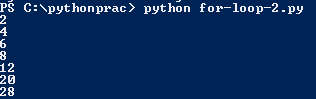In later examples, we’ll use for loops and if-else statements to create useful, complicated programs.

Nested For Loops

We nested loop is basically a loop within a loop. We covered a simple nested loop in an example above. Let’s go into this useful Python feature with a few more examples.

Suppose you have a list of items:

`                list1 = [“Cars”, “Aeroplanes”, “Ships”, “Bicycles”]`

To print out individual items from the list, you can use a simple for loop:

```                for i in list1:
print i```

This will yield the following output: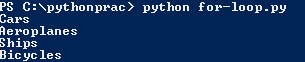But what happens if we add another for loop to the above loop?

Let’s find out:

```                for i in list1:
for x in i:
print x```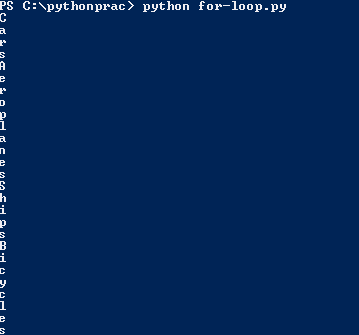Basically, Python breaks each word into its constituent letters and prints them out. This points out an important Python property – that strings are basically lists of individual alphabets.

It’s important to remember that you can nest any type of loops. A while loop can sit inside a for loop, which can sit inside an if-else conditional, and so on.

Exiting a For Loop

Sometimes, it’s necessary to implement a fail-safe mechanism to exit the loop in case some of the conditions of the loop aren’t met. This is usually required with while loops but can also be used with for loops. The proper method to do this in Python is using the break command.

Let’s see how it works:

```x = range(1, 100)
for i in x:
break
print i```

This will produce no output at all because you’ve commanded Python to break the program flow before it can print anything.

In examples below, we’ll learn how we can use Python for loops to create different programs. We’ll also learn about some Python commands and features we didn’t touch upon before.

Examples

1. Create a list of items using input provided by users

This is a great example of a simple, beginner program that uses for loops and lists. As with any programming exercise, it helps to list down the basic requirements before writing any code. In this program we want:

• Create an empty list.
• Ask the user how many items he wants to add to the list.
• Add said items to the list.
• Print items.

Based on these requirements, we can gather that we’ll need to use raw_input() to gather user input, and append() to add elements to the list.

So let’s get started!

```from sys import argv #Import command used to import argv or argument variable
script, user_name = argv #argv used to extract user name and program name
print "Hello, welcome to the %s program, %s" % (script, user_name)
print "This program will ask you to enter the names of places you've been to"
print "Before we get started, please enter the number of places you want to enter"
number = int(raw_input("> "))
print "Thank you!"
print "We'll now ask you to enter all the places you've traveled to"
places_traveled = [] #Empty list created to hold entered values
for i in range(number): #range(number) used because we want to limit number of inputs to user coice
places = raw_input("> ")
places_traveled.append(places)
print "Here's a list of places you've been to: \n", places_traveled```

Running the program, we see this (make sure that you enter your user name after the program name in command prompt):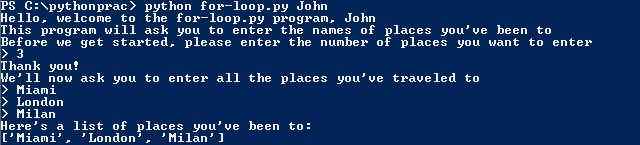Let’s break down and understand the program in a bit more detail:

`from sys import argv`

argv is called ‘argument variable’. It’s just like a normal variable, except that it’s called when the program is run in the command prompt itself. This can be very useful when you want to enter a user name or program name, as in the example above. In this program, we specifically used argv because we wanted to extract the name of the script.

`script, user_name = argv`

This line defines the two argument variables to be used in the program. Script, which is an in-built method, extracts the name of the program.

`number = int(raw_input(“> “))`

We enclosed raw_input() within int() because we want to make sure that the entered value is an integer, not a string. Try to run the program again and enter a word instead of the number at the first prompt. You’ll get the following error: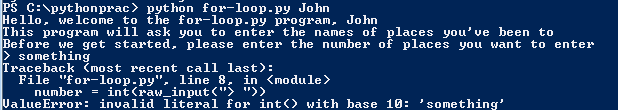This is Python telling you that it expected an integer here but got a string instead.

`places_traveled = []`

Yes, you can create empty lists as well!

`for i in range(number):`

Instead of declaring the range ourselves, we asked the user for the number of items he/she wants to enter. Hence, we declared the variable before using it in the loop.

This is a solid enough beginner program, but how can you improve it? Here are some homework questions you can work on:

1. We used int() to make sure that the entered input is a number. But is there any way to verify whether the input is a string?

Hint: Look up the isalpha() function in Python.

2. Expand the program to include more than one list – say, a list of places you’ve traveled to and the people you traveled with.

2. Joining strings

Although this exercise makes only a cursory use of for loops, we’ll use it to introduce a few useful Python functions, namely: isalpha(), len, and join().

The basic requirements are as follows:

• Create an empty list.
• Ask user to input strings into list.
• Verify whether data is valid, i.e. entered data is a string, not a number, and it is at least one character long.
• Print list with different formatting.

Here’s the complete program:

```from sys import argv
script, user_name = argv
print "Welcome to the %s script, %s" % (script, user_name)
print "In this program, we'll ask you to enter a few pieces of text"
print "We will then verify whether the input is correct or not"
print "Finally, we'll display the contents of the list with different formatting"
print "So let's get started!"
list1 = []
for i in range(3):
item = raw_input("Please enter a text item > ")
if len(item) > 0 and item.isalpha():
list1.append(item)
else:
print "You entered an invalid input!"
print "Remember to enter text input only!"
print "This is what your final list looks like", list1
print "Let's see how the list looks with different formatting"
print " ".join(list1)
print "--".join(list1)
print "...".join(list1)
print ", ".join(list1)```

Here’s the result of the program: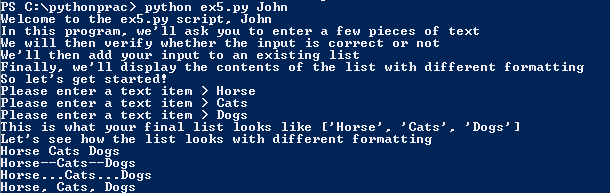Let’s try to understand what’s going on here:

`if len(item) > 0 and item.isalpha():`

Here, we are using two built-in Python functions – len() and isalpha() to check for valid input. Len() returns the length of the mentioned variable. Here, we want the entered data to be at least one character long. Hence, we’ve used len(item) > 0.

isalpha() is another built-in Python function that checks whether a variable contains only alphabets or not. item.isalpha() essentially means: “proceed only if the entered input is alphabetical. If it contains any numbers, move on to the else statement”.

Try it out right now – rerun the program, but use a number in the first prompt instead. You’ll see the following output: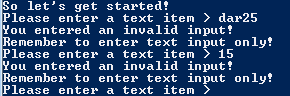A combination of len() and isalpha() is great for checking user input. For example, you can use len() to check whether a user name or password field in a web form is empty, or contains too many characters.

The next interesting bit of code in the example is join:

```print " ".join(list1)
print "--".join(list1)
print "...".join(list1)
print ", ".join(list1)```

Here, join() is used to print out individual items in the list separated by the specified format. The format must be enclosed within quotes. In the first case, we’ve separated individual items by a space, in the second case, by two dashes, in the third, by three dots, and in the fourth, by a comma and a space.

For homework, try to complete the following exercise:

1. Look up both len() and isalpha() on official Python documentation. Can you think of any other situations where these two might be used?
2. We’ve used join(), but there’s another Python function called split() that can be used to break down strings. Look up split() on the official Python documentation. How does it differ from join()? What are some possible use cases for split()?

3. Printing first n numbers and checking whether they are prime

This is a great example that utilizes nested loops, if-else statements and the break command. The basic requirements are as follows:

• Create a list of n numbers
• Check whether a number is prime or not. Remember that a number is prime only if it has two divisors – one and itself.
• If a number is not prime, list its divisors.
• If a number is prime, print that ‘x is a prime number’.

Based on this information, we can start creating our program:

```for i in range(2, 20):
for x in range(2, i): #Using nested loops to get numbers within first range
if i % x == 0: #Checking whether first number is divisible by second number
print i, "equals", x, "*", i/x
break
else:
print i, "is a prime number"```

The result:For a newbie, the mathematical operation above might seem very complicated, so let’s break this down into more digestible chunks:

`for i in range(2, 20):`

This is used to get a number, i, that lies between the range 2-20.

`for x in range(2, i):`

Here, we get another number, x, that lies between the range 2 and i. Thus, if the first number, i, is 6, the range here would be 2-6.

`if i % x == 0:`

This is a check for divisibility. We know that if a number has more than two divisors, it is not prime. If the first number, i, is perfectly divisible by the second number, x, it means it has at least three divisors – itself, 1, and x.

`print i, "equals", x, "*", i/x`

This is a simple print statement that lists the number i and its divisor, x.

`break`

We used break here because we want the program to proceed to the else statement instead of going through the for loop again. If we remove break, we get the following output: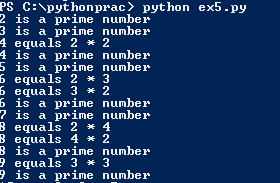This is clearly incorrect as 4, 6, 8 and 9 are not prime numbers.

```else:
print i, “is a prime number”```

Here, we’ve used an else clause in a for loop. The else conditional isn’t limited to if-else statements alone. You can use it with a for loop as well, provided you’ve used a break in the for loop somewhere. Using else essentially tells Python to switch to the code under else after it encounters a break. Thus, in the above example, we are instructing Python to break the code if the first number is divisible by the second number, and check the code under else instead.

For homework, answer the following questions:

1. In plain English, write down what Python is doing for each line in the code.
2. List the program ‘flow’. Pick a number, say, 6, and see what happens when i = 6.
3. Look up else clauses in Python documentation online.

Q. Where should you use a for loop instead of a while loop?

Almost everywhere. The while loop has its uses, but it is also prone to throw off errors. As a beginner, try to minimize the number of while loops in your programs and stick to for loops instead. Sure, there are problems where you can’t help but use a while loop, but if possible, stick to for loops – whether you’re printing a list of numbers, working with lists, or making decisions with if-else statements.

Q. How many for loops can I nest inside existing loops?

As many as you want (or as many as the program requires). But like if-else statements, it’s best to limit for loops to two levels deep. Deeper than that and you’ll start walking towards ‘bad practice’ territory (and other programmers won’t like you anymore).

Q. I saw a ‘pass’ command in some code online. What is that?

A ‘pass’ command is basically an empty placeholder. It doesn’t do anything, but is used in a place where some code is required syntactically. For an example, look at the question below.

Q. What is the difference between range and xrange?

If you looked up range online, you may have encountered xrange as well. xrange is another way to specify range when using a for loop. It works exactly like range, except that it is faster and more memory efficient.

When you use range, you essentially create a list which Python reiterates through. Thus, for x in range(0, 100) basically creates a temporary list with numbers from 0 to 100. As the for loop is executed, Python goes through each element in this list. This can be slow, especially when dealing with very large range of values.

xrange on the other hand, generates an object that creates the number(s) required in the range. Understanding how this works requires going into object-oriented programming, which is an entirely new ball game altogether.

You can test the runtime of range (or any other function) and xrange by using the timeit module. To use this method, enter the following code in the command prompt:

`python –m timeit ‘for x in range(0,200000):’ ‘ pass’`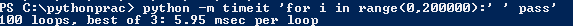(Notice how we used ‘pass’ here because the for loop required an indented block syntactically, but the program didn’t)

Doing the same for xrange: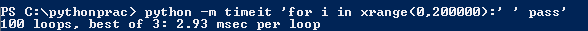Thus, xrange reiterates through the range in 2.93 msec per loop, while range does the same in 5.95 msec per loop, making xrange nearly twice as fast as range.

Q. What are dictionaries?

We briefly mentioned dictionaries in this tutorial. Dictionaries are basically another type of sequence in Python. They’re list lists, except that they also have a key and a value associated with it. You can think of them like actual English dictionaries, where there are words (keys) and a meaning (value) associated with the word.

The basic syntax of a dictionary is as follows:

`                dictionary = {key1: value1, key2: value2, key3: value3}`

Here’s an example:

`                animals = {“rabbit”: “carrots”, “cow”: “grass”, “lion”: “meat”}`

Here, ‘rabbit’, ‘cow’, and ‘grass’ are the keys, while ‘carrots’, ‘grass’, and ‘meat’ are the values. Each key can have multiple values as well:

`                animals = {“rabbit”: (“carrots”, “cabbage”, “cucumber”), “cow”: “grass”, “lion”: “meat”}`

You can print any particular value from the dictionary by mentioning its key:

`                print animals[“rabbit”]`

This will print (‘carrots’, ‘cabbage’, ‘cucumber’).

But what if you want to print both keys and values? To do this, you’ll have to use a for loop and a built-in function called iteritems().

```                for i, value in animals.iteritems():
print i, value```

Here, both i and value are arbitrary placeholder variables. You can use any other variable that you like. The result would look like this: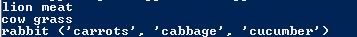Dictionaries can be very useful for storing data. Imagine a dictionary that stores a user’s name, password, login, age, and gender which you can use to extract and display specific data.

You can learn more about dictionaries and other intermediate level Python topics in this course on Python for beginners.

The best place to learn Python syntax and built-in functions is by going through the official Python documentation at docs.Python.org. Another option is to simply Google ‘Python + [command or function]’. You can also get an overview of specific Python functions and features through the built-in pydoc. Just type in the following at the command prompt:

`                python –m pydoc command-name`

Here’s an example for open():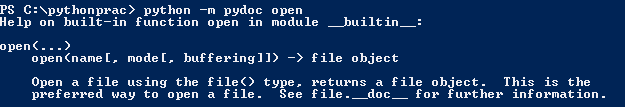Q. Where can I find Python programs and code?

Programming languages are a lot like actual languages. You can sit in a classroom and learn hundreds of words and grammar rules, but the only way to master a language is by actually using it in your daily life, preferably in a country where it’s spoken natively. The same rule applies to Python and other programming languages. Hang out at websites like StackOverflow.com and GitHub.com to see how other programmers use the language and get access to sample code. GitHub hosts thousands of code libraries and software source code in Python and is a great place to learn the language.

Next Steps

You are now familiar with the most frequently used loop in Python, the for loop. Your next steps after this tutorial is should be to familiarize yourself with the while loop, dictionaries, and eventually dictionaries, classes and object-oriented programming. Try some of these courses below to get started:

Page Last Updated: November 2013

### Top courses in Python

Learn Python & Ethical Hacking From Scratch
Zaid Sabih, z Security
4.7 (14,219)
REST APIs with Flask and Python
Jose Salvatierra, Teclado by Jose Salvatierra
4.6 (14,807)
Bestseller
Spark and Python for Big Data with PySpark
Jose Portilla
4.6 (16,070)
Bestseller
The Complete Python Course | Learn Python by Doing
Codestars by Rob Percival, Jose Salvatierra, Rob Percival, Teclado by Jose Salvatierra
4.6 (15,248)
Taming Big Data with Apache Spark and Python - Hands On!
Sundog Education by Frank Kane, Frank Kane, Sundog Education Team
4.5 (11,256)
Data Analysis with Pandas and Python